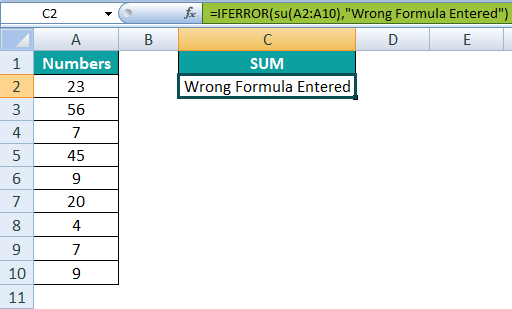# IFERROR In ExcelArticle byExcelMojo Team## What Is IFERROR Excel Function?

IFERROR Excel Function helps users find and manage errors in formulas and calculations. The function checks the formula, and if a mistake is found, it returns another specified value. Otherwise, the formula returns the result. The errors handled by the function are #N/A, #VALUE!, #REF!, #DIV/0!, #NUM!, #NAME?, or #NULL!.

For example, cells A1 and A2 contain 240 and 78, while cells B1 and B2 have 60 and 0, respectively. We perform the following tasks in the given sequence:

1. =IFERROR(A1/B1,”Error in Calculation”) – The error is checked by dividing 240/60. If it finds no error, it will return the result as 4.
2. =IFERROR(A2/B2,”Error in Calculation”) – The error is checked by dividing 78/0. Since it will find 0 after division, it will return “Error in Calculation.”

In this way, the IFERROR Excel formula replaces the error message (#DIV/0!) with a customized text string (“Error in Calculation”).

###### Key Takeaways

• The IFERROR function handles many types of errors like the #NUM, #VALUE etc. and returns a specified message if these errors are found.
• If a formula returns an error, we ca use the IFERROR to return an alternative result.
• The IFERROR formula in Excel is: =IFERROR(value, value_if_error). Here, value checks for errors in the conditions while the second argument returns the value if there is an error.

### IFERROR() Excel Formula

• valueThis is the value to be checked for errors. It can be a cell reference, formula, logical value, or expression. It is a required argument in the formula.
• value_if_errorThe value is returned if the formula finds an error. It can be text, number, logical value, etc. It is also a required argument in the formula.

The IFERROR function evaluates the following error types in Excel:

### How To Calculate IFERROR In Excel?

Let us look at some IFERROR functions in Excel examples to understand the formula:

#### Example #1 – Using With SUM

Here is how you can use IFERROR excel function. Suppose there are three values in cells C2, C3, and C4, as 10, 20, 30, and the sum is calculated.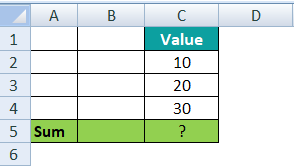1. Sum all three values 10, 20, 30 using the following formula in cell C5:

=C2+C3+C4 = 60

But, instead of the “+”, the operator enters a space by mistake.2. The error generated by entering an invalid formula is #NULL!.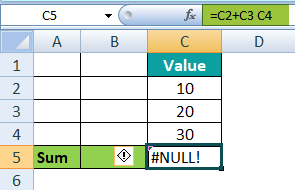3. Using the IFERROR function, the error made can be corrected. The following IFERROR Excel formula is entered in cell C5:

=IFERROR(C2+C3 C4,SUM(C2:C4))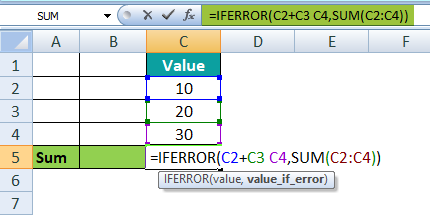4. The IFERROR function generates the result as 60.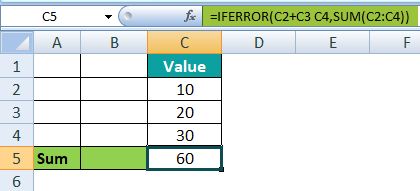#### Example #2 – IFERROR With VLOOKUP

The following image shows the salary details of every employee of a company. Using the IFERROR VLOOKUP function, we will try to understand the #N/A error in the following example.

In the table, the data is reflected as below:

• Column A contains the ID of employees
• Column B contains the Name of employees
• Column C contains the Salary of employees

We fetch the value from cell E2 to F3 using the VLOOKUP function.

Step 1: Perform the VLOOKUP function in cell F3. Search the salary of the employee whose ID is 20.

VLOOKUP function in Excel will look up the value in the first column:

=VLOOKUP(F2,A2:C6,3,FALSE)

Step 2: The error #N/A is found since no employee has the ID 20 in the table array.

Step 3: Replace the #N/A error with the “Value Not Found” text string. The following formula is entered in cell F3:

Step 4: The VLOOKUP function does not evaluate errors in the formula. Therefore, the IFERROR function returns the “Value Not Found” text string.

Hence, the text string replaces the ‘#N/A’ error with the ‘Value Not Found.’

#### Example #3 – IFERROR Function Returns 0 In Case Of Error

The following image shows the inventory of the vegetable vendor. Using the IFERROR function, we will try to understand the #DIV/0! error in the following example:

In the table, the data is reflected as below:

• Column A contains the name of Veggies
• Column B contains the Price of veggies
• Column C contains the Total Quantity of veggies
• Column D contains Prices As Per Quantity to be calculated

Step 1: Perform the division function in column D. Calculate price as per quantity using the following formula. But, the result of cucumber in cell D4 generates an error as “#DIV/0!” because the price is divided by 0.

=B2/C2

Step 2: Replace the #DIV/0! error with the numeric value 0. The following formula is entered in cell D2:

=IFERROR(B2/C2,0)

Step 3: The IFERROR function returns the numeric value “0” in the place of the DIV/0! error.

Press the “Enter” key. Then, drag the formula downwards till cell D7. The output of the #DIV/0! error is replaced with 0 in the following image:

=IFERROR(B4/C4,0)

#### Example #4 – Handling #VALUE Error

The following image shows the calculation of the monthly salary of employees according to the hourly rate, hours worked and paid hours off. Using the IFERROR function, we will try to understand the #VALUE! error in the following example:

In the table, the data is reflected as below:

• Column A contains Employee Names
• Column B contains the Hourly Rate
• Column C contains Hours Worked
• Column D contains Paid Hours Off
• Column E calculates the Monthly Salary

Step 1: Monthly salary is calculated using the following formula in cell E2 and dragging it down to cell E9 for the same calculation.

=(C2+D2)*B2

Step 2: In the two cells, E4 and E8, the #VALUE! error is generated.

Step 3: To replace the #VALUE! error IFERROR function is used. The following formula is used to correct the error generated:

=IFERROR((C2+D2*B2,B2*D2)

Step 4: The IFERROR function returns the solution in the place of the #VALUE! error.

Press the “Enter” key. Then, drag the formula downwards till cell E9. The output of the #VALUE! error is replaced in the following image.

=IFERROR((C4+D4)*B4,B4*D4)

### IFERROR Best Practices

• Do not trap error without any reason – The error can be identified using the IFERROR function in Excel, but this does not mean that one should wrap every formula with error handling.
• Wrap the smallest possible part of a formula in IFERROR – Use the error handling function of smaller scope.
• Use specific error handling functions:
• IFNA or ISNA function in Excel is the simplest way to catch “#N/A” errors.
• ISERR function in Excel can catch all errors except “#N/A”.

What is IFERROR?

The IFERROR function handles many Excel errors, like #N/A, #VALUE!, #REF!, #DIV/0!, #NUM!, #NAME?, or #NULL!. The IFERROR function returns the value if it finds these errors. These values can be text, number, empty cell, logical value or expression, etc.

– The IFERROR function is used to catch errors in a formula.
– The IFERROR function notifies the user about replacing the error with the specified message.

What is IFERROR formula in Excel?

The IFERROR formula in Excel is:

=IFERROR(value,value_if_error)

The following formula arguments are mandatory:

– The first argument is “value, which checks for errors in the given condition.
– The second argument is value_if_error”, which returns the value if the first argument detects the error.

How does IFERROR work in Excel?

The IFERROR function in excel works by using the following formula:

=IFERROR(value,value_if_error)

For example, there is a list of numbers, and the sum of those numbers is to be calculated.

In the table, the data is reflected as below:

Column A contains Numbers
Column C calculates the Sum

Here, the wrong formula of the sum is entered. For example, the entered formula is su(A2:A10), but it should be SUM(A2:A10). Due to this, #NAME? error is generated.We will replace the #NAME? error with the ‘Wrong Formula Entered’ text string by entering the following formula in cell C2:

=IFERROR(su(A2:A10),”Wrong Formula Entered”)

Hence, the IFERROR function replaces the #NAME? with the ‘Wrong Formula Entered’ text string.